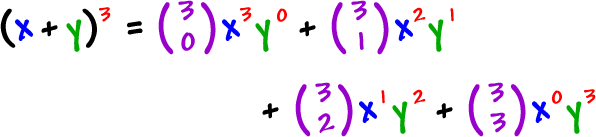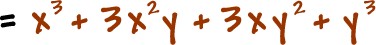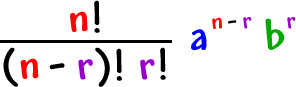Look familiar?

Dude!  It's Pascal's Triangle?

Those "choose" things make the numbers in Pascal's Triangle!  I think that's pretty dang cool!

What did we use Pascal's Triangle for?

The coefficients of binomial expansions!

So, we can be a LOT more efficient.

Let's crunch an example and you'll see how it works:It's even easier to see what's going on when we write out the coefficients:Here's the form for ONE of these guys:

 For the expansion of